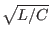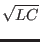Next: Initial Conditions Up: Transmission Lines (General) Previous: Model Level   Contents   Index

### Electrical Characteristics

len
This provides the physical length of the transmission line in arbitrary units, though the units must match the per-length unit in the element values discussed below. If not given, the value is taken as unity, unless it is implicitly defined by other parameters.

l
This parameter provides the series inductance per unit length of the line. The default is 0.

c
This parameter provides the shunt capacitance per unit length of the line. The default is 0.

r
This parameter provides the series resistance per unit length of the line. The default is 0.

g
This parameter provides the shunt conductance per unit length of the line. The default is 0. With level 2, this cannot be nonzero if l or c is given, i.e., only r can be nonzero if g is nonzero for level = 2, as in the SPICE3 LTRA model.

z0 or zo
This is the line (lossless) characteristic impedance in ohms, given by

Z0 =td or delay
This is the (lossless) phase delay of the line in seconds, given by

Td = Lengthnl
This is the normalized line length at a particular frequency f, which must also be specified (see below). This is an alternative means for setting the line delay, where

Td = nl /f

It is an error to give both td and nl.

f
This is the frequency at which the normalized line length (above) is representative.

To model a line with nonzero series inductance and shunt capacitance, a complete but non-conflicting subset of the parameters l, c, z0, td, len, f, and nl must be provided. The td parameter is the line delay in seconds, and the z0 parameter is the impedance in ohms, for the lossless case. Specifying these two parameters is sufficient to completely specify a lossless line, or the reactive elements of a lossy line. Alternatively, one could specify l (inductance per length), c (capacitance per length) and len (line length). If len is not specified in either case, the length defaults to unity. The delay can also be specified through the f (frequency) and nl (normalized length) parameters, where the delay would be set to nl/f. It is an error to specify both td and f, nl. If td is specified, or both f and nl are specified, along with parameters which yield internally the L and C values, then the length is determined internally by

Length = Td/One can specify z0 and l, for example, which determines C. Unlike the SPICE3 (and SPICE2) lossless line devices, the delay must be specified through the parameters; there is no default.Next: Initial Conditions Up: Transmission Lines (General) Previous: Model Level   Contents   Index
Stephen R. Whiteley 2022-09-18